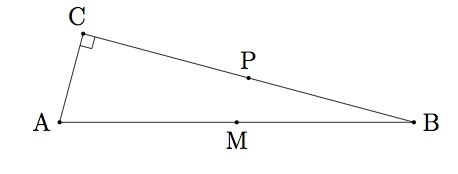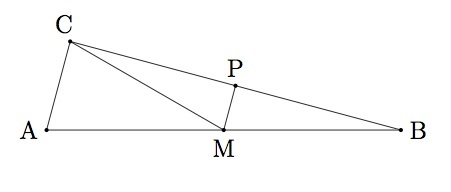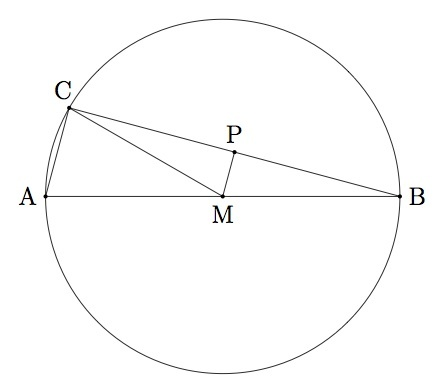# Right triangles inscribed in circles II

Alignments to Content Standards: G-C.A.2

Suppose $ABC$ is a triangle with angle $ACB$ a right angle. Suppose $M$ is the midpoint of segment $\overline{AB}$ and $P$ the midpoint of segment $\overline{BC}$ as pictured below:1. Show that angle $MPB$ is a right angle.
2. Show that triangle $MPB$ is congruent to triangle $MPC$.
3. Conclude from (b) that triangle $ABC$ is inscribed in the circle with diameter $\overline{AB}$ and radius $|MA|$.

## IM Commentary

The result here complements the fact, presented in the task ''Right triangles inscribed in circles I,'' that any triangle inscribed in a circle with one side being a diameter of the circle is a right triangle. A second common proof of this result rotates the triangle by $180$ degrees about $M$ and then shows that the quadrilateral, obtained by taking the union of these two triangles, is a rectangle. The basic steps in this alternative argument are:

• This quadrilateral can be shown to be a parallelogram because of the $180$ degree rotations used in its construction.
• Its diagonals are congruent (since one is a rotation of the other).
• A parallelogram with congruent diagonals must be a rectangle.

If used for instructional purposes and if time permits, the teacher may wish to discuss this alternate approach.

The approach taken here is more straightforward and relies mainly on the result saying that the line through the midpoints of two sides of a triangle is parallel to the line containing the third side. Due to its dependence on this result the task is mainly intended for instruction. In the absence of this result, part (a) will be difficult though an alternate solution is sketched. The task can also be made more open ended and challenging by omitting part (b).

## Solutions

Solution: 1

1. Since line $\overline{MP}$ joins the midpoints of two sides of a triangle, it follows that line $\overline{MP}$ is parallel to the line containing the third side of the triangle, namely line $\overline{AB}$. Since $\overleftrightarrow{AB}$ is perpendicular to $\overleftrightarrow{BC}$ and $\overleftrightarrow{MP}$ is parallel to line $\overleftrightarrow{AB}$, it follows that $\overleftrightarrow{MP}$ is also perpendicular to $\overleftrightarrow{AB}$. Hence angles $MPB$ and $MPC$ are both right angles.

2. Below are pictures of the triangles $MPB$ and $MPC$ which will be shown to be congruent:By part (a), angles $MPB$ and $MPC$ are congruent because they are both right angles. Since $P$ is the midpoint of segment $\overline{BC}$ we know that segment $\overline{PB}$ is congruent to segment $\overline{PC}$. Since segment $\overline{MP}$ is congruent to itself, it follows from SAS that triangle $MPB$ is congruent to triangle $MPC$.

3. From part (b), segment $\overline{MC}$ is congruent to segment $\overline{MB}$ because these are corresponding sides of congruent triangles $MPB$ and $MPC$. Since $M$ is the midpoint of segment $\overline{AB}$ it follows that $\overline{MA}$ is congruent to $\overline{MB}$. Since $|MC| = |MB|$ and $|MA| = |MB|$ it follows that $|MA|, |MB|$, and $|MC|$ are all equal. Therefore the circle with center $M$ and radius $|MA|$ passes through all three points $A,B,$ and $C$. Since all three vertices of triangle $ABC$ are on this circle, the triangle is inscribed in this circle. Below is a picture showing the circle in which triangle $ABC$ is inscribed:Solution: 2 Alternate solution for part (a)

The triangles $ACB$ and $MPB$ can be shown to be similar using the $SAS$ Similarity Theorem:

• Angle $B$ is shared by the two triangles.
• We are given that $$\frac{|CB|}{|PB|} = \frac{|AB|}{|MB|} = \frac{1}{2}.$$

The $SAS$ Similiary Theorem implies that triangles $ACB$ and $MPB$ are congruent. Since angle $C$ is a right angle, it follows that angle $MPB$ is also a right angle.